# Pythagorean theorem - examples - page 9

1. Diagonals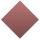Rhombus has two diagonals e=14 dm and f=11 dm. Calculate the side angle and height of the rhombus.
2. CarpetThe room is 10 x 5 meters. You have the role of carpet width of 1 meter. Make rectangular cut of roll that piece of carpet will be longest possible and it fit into the room. How long is a piece of carpet? Note .: carpet will not be parallel with the diag
3. OPT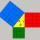What is the perimeter of a right triangle with the legs 14 cm and 21 cm long?
4. CenterCalculate the coordinates of the circle center: ?
5. Prism - boxThe base of prism is a rectangle with a side of 7.5 cm and 12.5 cm diagonal. The volume of the prism is V = 0.9 dm3. Calculate the surface of the prism.
6. RhombusCalculate the length of the diagonal AC of the rhombus ABCD, if its perimeter is 84 dm and the other diagonal BD has length 20 dm.
7. Above EarthTo what height must a boy be raised above the earth in order to see one-fifth of its surface.
8. Median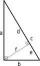In the right triangle are sides a=41 dm b=42 dm. Calculate the length of the medians tc to the hypotenuse.
9. Common chordTwo circles with radius 17 cm and 20 cm are intersect at two points. Its common chord is long 27 cm. What is the distance of the centers of these circles?
10. Vertical prismThe base of vertical prism is a right triangle with leg a = 5 cm and a hypotenuse c = 13 cm. The height of the prism is equal to the circumference of the base. Calculate the surface area and volume of the prism
11. Leg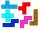Determine the area of a trapezoid with bases 71 and 42 and height is 4 shorter than the its leg.
12. Chord ABWhat is the length of the chord AB if its distance from the center S of the circle k(S, 92 cm) is 10 cm?
13. Isosceles trapezoidThe lengths of the bases of the isosceles trapezoid are in the ratio 5:3, the arms have a length of 5 cm and height = 4.8 cm. Calculate the circumference and area of a trapezoid.
14. Segment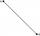Calculate the length of the segment AB, if the coordinates of the end vertices are A[10, -4] and B[5, 5].
15. TetrahedronCalculate height and volume of a regular tetrahedron whose edge has a length 18 cm.
16. Trapezoid RTThe plot has a shape of a rectangular trapezium ABCD, where ABIICD with a right angle at the vertex B. side AB has a length 36 m. The lengths of the sides AB and BC are in the ratio 12:7. Lengths of the sides AB and CD are a ratio 3:2. Calculate consumptio
17. Rectangular trapezoidThe rectangular trapezoid ABCD is: /AB/ = /BC/ = /AC/. The length of the median is 6 cm. Calculate the circumference and area of a trapezoid.
18. Goniometric form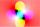Determine goniometric form of a complex number ?.
19. Center of the cubeCenter of the cube has distance 33 cm from each vertex. Calculate the volume V and surface area S of the cube.
20. TriangleCalculate the sides of the triangle if its area S = 630 and the second cathethus is shorter by 17.

Do you have an interesting mathematical example that you can't solve it? Enter it, and we can try to solve it.

To this e-mail address, we will reply solution; solved examples are also published here. Please enter e-mail correctly and check whether you don't have a full mailbox.

Pythagorean theorem is the base for the right triangle calculator.